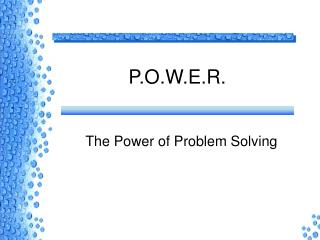DownloadDownload PresentationP.O.W.E.R.

# P.O.W.E.R.

Download Presentation## P.O.W.E.R.

- - - - - - - - - - - - - - - - - - - - - - - - - - - E N D - - - - - - - - - - - - - - - - - - - - - - - - - - -
##### Presentation Transcript

1. P.O.W.E.R. The Power of Problem Solving

2. Perceive • Apprehend • decipher, • deduce, • discern, • discover, • distinguish, • experience, • gather, • grasp, • identify, • know, • read, • understand

3. Perceive • How? – Ask Questions • What can I infer from this information ? • You mean that if I know…, then that means…)

4. Perceive • What if… • Imagine a change in the hypothesis or given information • Imagine an action on the given data. • What information do I know that will help me do this problem> • What is the basic question in this problem?

5. Organize • Organize information in a table • Draw a picture • Look for patterns • List given information • What is the main question?

6. Work the Problem • Write an equation and solve • Simplify • Evaluate • Graph • Find and interpret data • Follow a procedure

7. Examine • Determine whether your result answers the basic question in the problem

8. Review • Ask how this problem is similar or different from other problems • Determine what kind of question could be asked on the test.